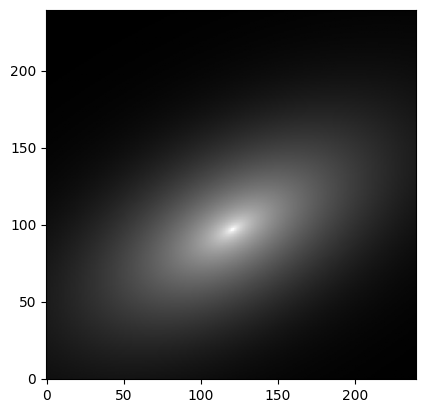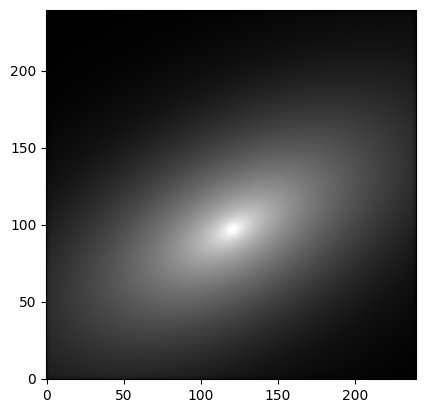# Tutorial / How to use¶

In this tutorial we create a (simplified) synthetic galaxy image from scratch, along with its associated segmentation map, and then run the statmorph code on it.

## Setting up¶

We import some Python packages first. If you are missing any of these, please see the the Installation section of the README.

:

import numpy as np
import matplotlib.pyplot as plt
import scipy.ndimage as ndi
from astropy.visualization import simple_norm
from astropy.modeling import models
from astropy.convolution import convolve
import photutils
import time
import statmorph
%matplotlib inline


Creating a model galaxy image

We assume that the image size is 240x240 pixels, and that the “true” light distribution is described by a 2D Sersic profile with the following parameters:

:

ny, nx = 240, 240
y, x = np.mgrid[0:ny, 0:nx]
sersic_model = models.Sersic2D(
amplitude=1, r_eff=20, n=2.5, x_0=120.5, y_0=96.5,
ellip=0.5, theta=-0.5)
image = sersic_model(x, y)
plt.imshow(image, cmap='gray', origin='lower',
norm=simple_norm(image, stretch='log', log_a=10000))

:

<matplotlib.image.AxesImage at 0x7fc4cfd304d0>Convolving with a PSF

In practice, every astronomical image is the convolution of a “true” image with a point spread function (PSF), which depends on the optics of the telescope, atmospheric conditions, etc. Here we assume that the PSF is a simple 2D Gaussian distribution:

:

size = 20  # on each side from the center
sigma_psf = 2.0
y, x = np.mgrid[-size:size+1, -size:size+1]
psf = np.exp(-(x**2 + y**2)/(2.0*sigma_psf**2))
psf /= np.sum(psf)
plt.imshow(psf, origin='lower', cmap='gray')

:

<matplotlib.image.AxesImage at 0x7fc4cfbbf690>Now we convolve the image with the PSF.

:

image = convolve(image, psf)
plt.imshow(image, cmap='gray', origin='lower',
norm=simple_norm(image, stretch='log', log_a=10000))

:

<matplotlib.image.AxesImage at 0x7fc4cfb60450>Here we add homogeneous Gaussian background noise, optimistically assuming that the signal-to-noise ratio (S/N) is 100 at the effective radius (where we had defined the Sérsic profile amplitude as 1.0). For simplicity, we do not consider Poisson noise associated with the source itself.

:

np.random.seed(1)
snp = 100.0
image += (1.0 / snp) * np.random.standard_normal(size=(ny, nx))
plt.imshow(image, cmap='gray', origin='lower',
norm=simple_norm(image, stretch='log', log_a=10000))

:

<matplotlib.image.AxesImage at 0x7fc4cfad1a50>Gain and weight maps

The code will ask for one of two input arguments: (1) a weight map, which is a 2D array (of the same size as the input image) representing one standard deviation at each pixel value, or (2) the gain, a scalar that can be multiplied by the science image to obtain the number of electron counts per pixel. The gain parameter is used internally by statmorph to calculate the weight map.

Here we assume, also somewhat optimistically, that there is an average of 10,000 electron counts/pixel at the effective radius (where we had defined the amplitude as 1.0), so that the gain is 10,000.

:

gain = 10000.0


Creating a segmentation map

Besides the image itself and the weight map/gain, the only other required argument is the segmentation map, which labels the pixels belonging to different sources. It is usually generated by specialized tools such as SExtractor, but here we create it using photutils:

:

threshold = photutils.detect_threshold(image, 1.5)
npixels = 5  # minimum number of connected pixels
segm = photutils.detect_sources(image, threshold, npixels)


Although statmorph is designed to process all the sources labeled by the segmentation map, in this example we only focus on the main (largest) source found in the image.

:

# Keep only the largest segment
label = np.argmax(segm.areas) + 1
segmap = segm.data == label
plt.imshow(segmap, origin='lower', cmap='gray')

:

<matplotlib.image.AxesImage at 0x7fc4cea64c90>We regularize a bit the shape of the segmentation map:

:

segmap_float = ndi.uniform_filter(np.float64(segmap), size=10)
segmap = segmap_float > 0.5
plt.imshow(segmap, origin='lower', cmap='gray')

:

<matplotlib.image.AxesImage at 0x7fc4ce9cac50>## Running statmorph¶

Measuring morphological parameters

Now that we have all the required data, we are ready to measure the morphology of the source just created. Note that we include the PSF as a keyword argument. In principle, this results in more correct Sersic profile fits, although it can also make the code run slower, depending on the size of the PSF.

:

start = time.time()
source_morphs = statmorph.source_morphology(
image, segmap, gain=gain, psf=psf)
print('Time: %g s.' % (time.time() - start))

Time: 0.825354 s.


In general, source_morphs is a list of objects, each corresponding to a labeled source in the image. Here we focus on the first (and only) labeled source.

:

morph = source_morphs


Now we print some of the morphological properties just calculated:

:

print('xc_centroid =', morph.xc_centroid)
print('yc_centroid =', morph.yc_centroid)
print('ellipticity_centroid =', morph.ellipticity_centroid)
print('elongation_centroid =', morph.elongation_centroid)
print('orientation_centroid =', morph.orientation_centroid)
print('xc_asymmetry =', morph.xc_asymmetry)
print('yc_asymmetry =', morph.yc_asymmetry)
print('ellipticity_asymmetry =', morph.ellipticity_asymmetry)
print('elongation_asymmetry =', morph.elongation_asymmetry)
print('orientation_asymmetry =', morph.orientation_asymmetry)
print('rpetro_circ =', morph.rpetro_circ)
print('rpetro_ellip =', morph.rpetro_ellip)
print('rhalf_circ =', morph.rhalf_circ)
print('rhalf_ellip =', morph.rhalf_ellip)
print('r20 =', morph.r20)
print('r80 =', morph.r80)
print('Gini =', morph.gini)
print('M20 =', morph.m20)
print('F(G, M20) =', morph.gini_m20_bulge)
print('S(G, M20) =', morph.gini_m20_merger)
print('sn_per_pixel =', morph.sn_per_pixel)
print('C =', morph.concentration)
print('A =', morph.asymmetry)
print('S =', morph.smoothness)
print('sersic_amplitude =', morph.sersic_amplitude)
print('sersic_rhalf =', morph.sersic_rhalf)
print('sersic_n =', morph.sersic_n)
print('sersic_xc =', morph.sersic_xc)
print('sersic_yc =', morph.sersic_yc)
print('sersic_ellip =', morph.sersic_ellip)
print('sersic_theta =', morph.sersic_theta)
print('sky_mean =', morph.sky_mean)
print('sky_median =', morph.sky_median)
print('sky_sigma =', morph.sky_sigma)
print('flag =', morph.flag)
print('flag_sersic =', morph.flag_sersic)

xc_centroid = 120.57974325587848
yc_centroid = 96.54876631751854
ellipticity_centroid = 0.49504651780554065
elongation_centroid = 1.980380441489651
orientation_centroid = -0.5025297323963465
xc_asymmetry = 120.49795192600573
yc_asymmetry = 96.5041141987735
ellipticity_asymmetry = 0.49503516784340607
elongation_asymmetry = 1.9803359289977076
orientation_asymmetry = -0.5025187596927418
rpetro_circ = 32.10584845059099
rpetro_ellip = 43.155342231457794
rhalf_circ = 14.607365607744995
rhalf_ellip = 19.197894171055044
r20 = 5.072220422468954
r80 = 26.11103164489153
Gini = 0.5674867473634592
M20 = -2.073215859383315
F(G, M20) = 0.2857979900017602
S(G, M20) = -0.05336512456445619
sn_per_pixel = 55.04891460404779
C = 3.5581295638595445
A = -0.0003891696891799785
S = 0.010867687703064226
sersic_amplitude = 1.0017449025553842
sersic_rhalf = 19.980728153552768
sersic_n = 2.496959225794045
sersic_xc = 120.50130875759518
sersic_yc = 96.50065293649406
sersic_ellip = 0.49994266664181736
sersic_theta = 2.6415533250460546
sky_mean = 0.00021039195094124476
sky_median = -1.9658131250737553e-06
sky_sigma = 0.010284385196536397
flag = 0
flag_sersic = 0


Note that the fitted Sersic profile is in pretty good agreement with the “true” Sersic profile that we originally defined (n=2.5, r_eff=20, etc.). However, such agreement tends to deteriorate somewhat at higher noise levels and larger Sersic indices (not to mention that real galaxies are not always well described by Sersic profiles).

Other morphological measurements that are more general and more robust to noise, which are also calculated by statmorph, include the Gini-M20 (Lotz et al. 2004), CAS (Conselice 2003) and MID (Freeman et al. 2013) statistics, as well as the outer asymmetry (Wen et al. 2014) and shape asymmetry (Pawlik et al. 2016).

Also note that statmorph calculates two different “bad measurement” flags (where 0 means good measurement and 1 means bad):

1. flag : indicates a problem with the basic morphological measurements.
2. flag_sersic : indicates if there was a problem/warning during the Sersic profile fitting.

In general, flag==0 should always be enforced, while flag_sersic==0 should only be used when interested in Sersic fits (which might fail for merging galaxies and other “irregular” objects).

Examining the fitted Sersic profile

Finally, we can reconstruct the fitted Sersic profile and examine its residual. Here we used the ConvolvedSersic2D class defined in statmorph.

:

ny, nx = image.shape
y, x = np.mgrid[0:ny, 0:nx]
fitted_model = statmorph.ConvolvedSersic2D(
amplitude=morph.sersic_amplitude,
r_eff=morph.sersic_rhalf,
n=morph.sersic_n,
x_0=morph.sersic_xc,
y_0=morph.sersic_yc,
ellip=morph.sersic_ellip,
theta=morph.sersic_theta)
fitted_model.set_psf(psf)  # required when using ConvolvedSersic2D
image_model = fitted_model(x, y)
bg_noise = (1.0 / snp) * np.random.standard_normal(size=(ny, nx))
fig = plt.figure(figsize=(15,5))
ax.imshow(image, cmap='gray', origin='lower',
norm=simple_norm(image, stretch='log', log_a=10000))
ax.set_title('Original image')
ax.imshow(image_model + bg_noise, cmap='gray', origin='lower',
norm=simple_norm(image, stretch='log', log_a=10000))
ax.set_title('Fitted model')
residual = image - image_model
ax.imshow(residual, cmap='gray', origin='lower',
norm=simple_norm(residual, stretch='linear'))
ax.set_title('Residual')

:

Text(0.5, 1.0, 'Residual')Examining other morphological diagnostics

For convenience, we also provide a make_figure function that can be used to visualize some of the basic morphological measurements carried out by statmorph. This creates a multi-panel figure analogous to Fig. 4 from Rodriguez-Gomez et al. (2019).

:

from statmorph.utils.image_diagnostics import make_figure
fig = make_figure(morph):

fig.savefig('tutorial.png', dpi=150)
plt.close(fig)